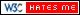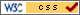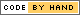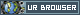# Vacuuming Robot

## Douglas Katz (djk289), Greg Cristina(gtc59)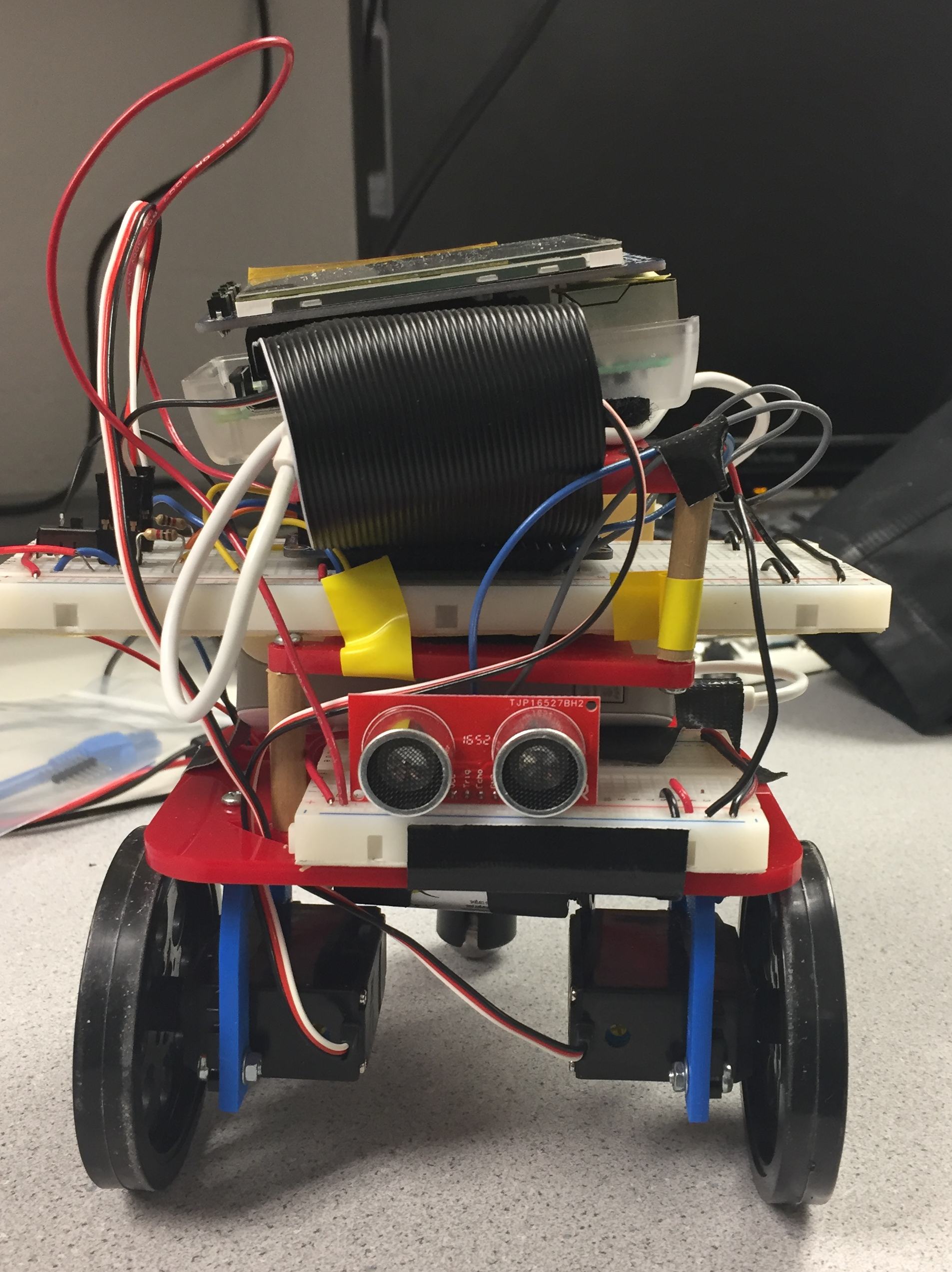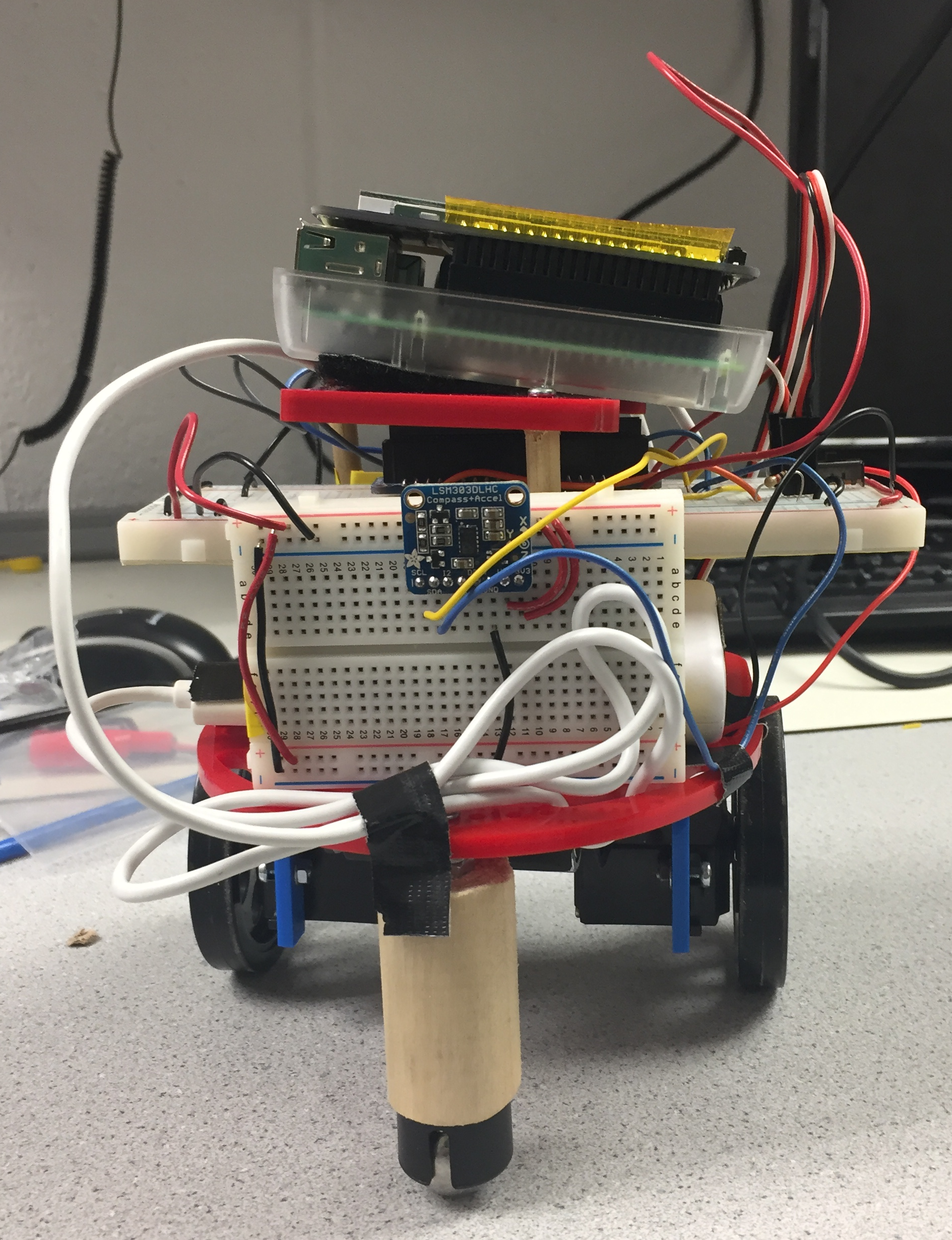## Objective

The objective of this project was to create a robot that would navigate through a room and clean up any dust or dirt on the floor. The robot was to use a vacuum or sweeper roller as its cleaning mechanism. The robot also aimed to cover the entire floor of a given room, such that the entire surface would be cleaned.

## Introduction

Our initial goal for this project was to create a robot that would clean a room autonomously, via a vacuum or sweeper setup, as stated in the project objective statement above. The robot would try to determine the size of the room by measuring how long it took to move along the outer edges of the room, and converting that time into distances, length and width. The robot would then pass through the room in a lawnmower pattern until the entirety of the room had been cleaned. While navigating, the robot would also display a map showing how much of the room had been cleaned. Once cleaning was finished the robot would stop moving, and a full map is displayed.

We were unable to attach a vacuum to the robot due to power constraints and we did not have time to design a frame to hold a roller that would brush debris into a bed. In the end we created a robot that could detect the size of a room and navigate through it completely. The robot was also able to display a map of the room in a grid format on a TFT display and would mark where it had been so far. Thus, the final result of the project became a room mapping robot rather than a room cleaning robot.

## Design and Testing

### Overview

The robot created for this project consisted of a few different subsystems. The first subsystem was the display which kept track of the room and used a TFT screen to display where the robot had been in the room. The second subsystem handled measuring the size of the room by using a distance sensor to detect walls, and measured how long it took to move across the dimensions of the room. The third subsystem controlled the movement and orientation of the robot. This subsystem used two servos attached to wheels to move the robot and used a magnetometer to aid the robot in maintaining a straight drive path.

### Display

The robot used a python class called arrayMap to handle keeping track of and currently displaying the robot’s past path. arrayMap would take in a room width, length, and the robot’s initial starting position post room dimension detection. It would then create a 2D room width by room length boolean array. The array acted as a grid of the room, where each space represented block of area the robot would cover. The block widths were about 5 inches, which matches the width of the robot. This dimension constraint would facilitate the robot to completely cover the entire room when moving through it. The spaces on the grid were all initially marked false. When the robot visited a space in the room, the corresponding space would be set to true. Once the entire grid had been marked true, the entire room had been visited or cleaned and the robot would stop. The way arrayMap was set up, it could only handle rectangular rooms.

The arrayMap class also contained a function for displaying the state of the grid on a small TFT display on the robot. The pygame library was used to in this function for displaying graphics on screen. The display function required two inputs called grid width and grid height that were equal to the dimensions of the display in pixels divided by the dimensions of the room. This allowed the robot to scale the grid to fit the display based on room dimensions. The display function then iterated through the arrayMap grid and drew two rectangles to the screen for each spot in the grid. The outer rectangle was of size grid width by grid height, followed by a second inner rectangle of a smaller size. The outer rectangle acted as a black border to the inner rectangle, which would turn white when that section of the grid was visited by the robot. This way, one viewing the screen could see individual grid blocks rather than one solid color. If the spot was not visited then the spot would be drawn in black and would not be visible. Figure 1 shows a diagram of the two rectangles and how they fit into a grid. Because the rectangles were sized based on the dimensions of the room, the display would also be filled completely when the robot made a full pass of the room. Figures 2 and 3 demonstrates the grid scaling capability.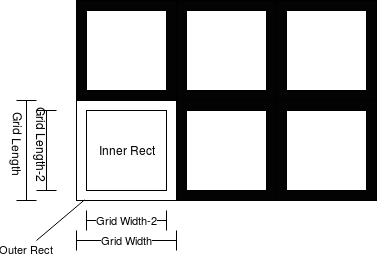Figure 1: arrayMap Rectangle Layout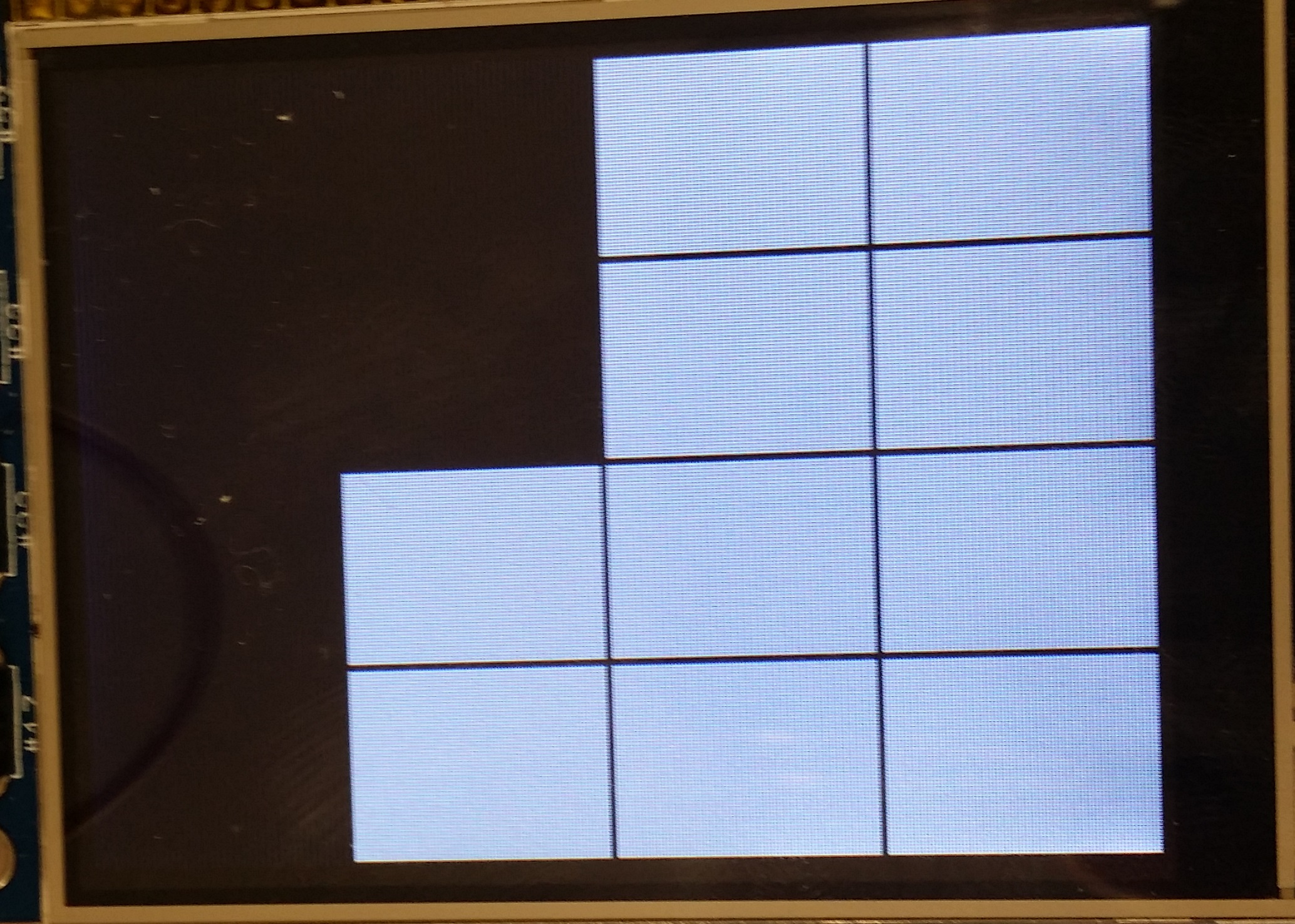Figure 2: 4x4 Grid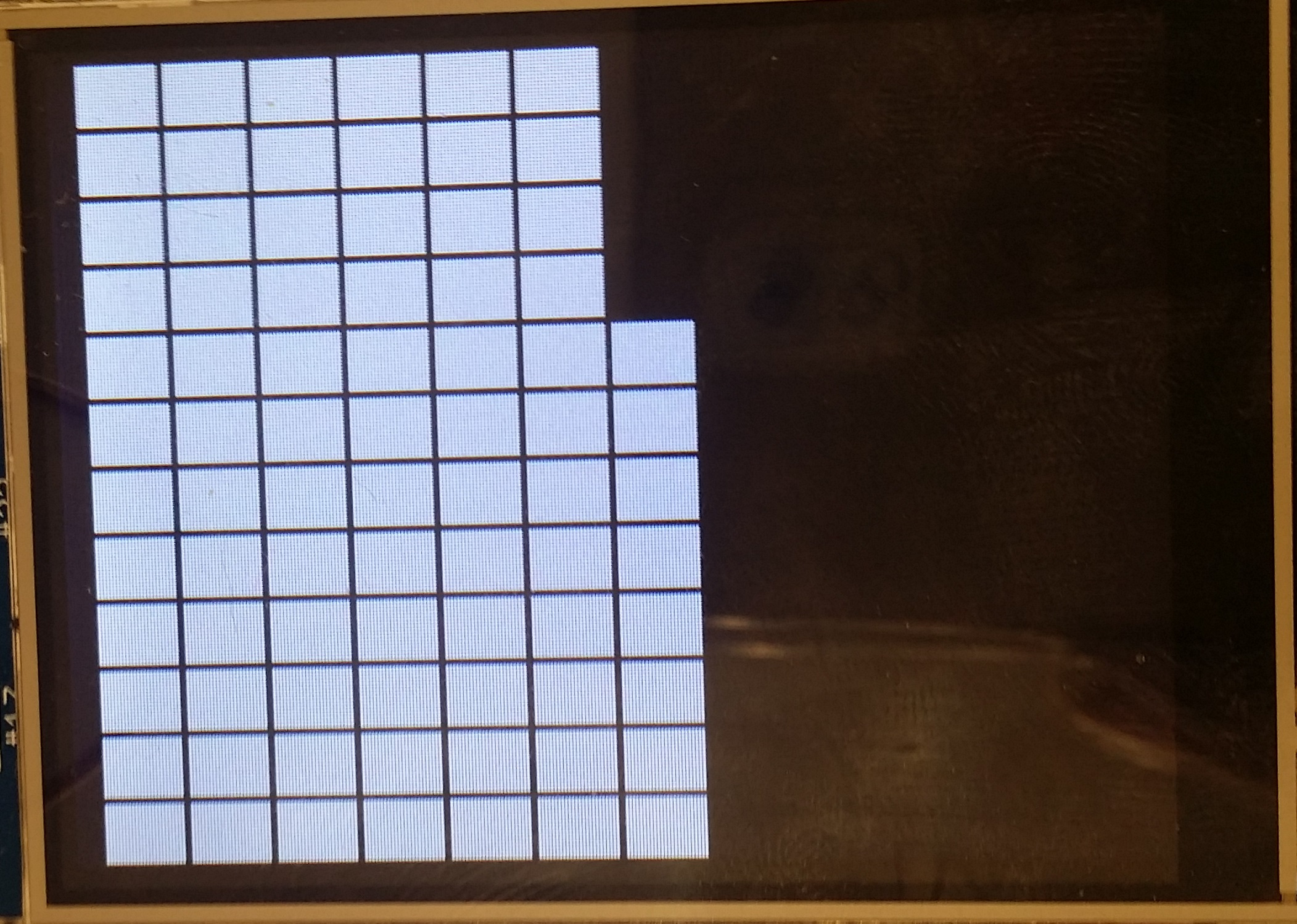Figure 2: 12x11 Grid

Testing the display began by testing the arrayMap class using the terminal for outputs. We printed the map to the terminal before and after making changes, i.e. adding blocks to the grid. This allowed for debugging before adding in pygame code. We created various testing functions for the class that allowed setting any space on the grid as visited or unvisited. We used this to ensure we were able to update spaces on the grid and print them correctly. Once we were satisfied we worked on added a move function to array class that allowed moving the current position on the grid north, south, east, or west different amounts. We tested moving around the grid and ensuring spots were marked visited as we moved to them. We also confirmed that it was not possible to move off the grid. Once we were satisfied that the map was working correctly we added pygame code so that the map could be displayed on a screen. We then testing moving around and checked if the screen would update correctly.

### Room Measurement

In order to know when it had finished navigating the entire room, the robot needed to be able to determine the size of the room it was in. We limited the rooms to being rectangular for simplicity, so the robot only needed to determine the width and length of the room to know the complete size. The robot began determining the size by moving up the room in 5 inch steps. After each step forward the robot would check if there was a wall in front of it using the distance sensor. If a wall was not in front of it, the robot took another step forward. If there was a wall then the robot knew it had reached the end of the room and counted the number of steps it had taken to reach the wall and multiplied it by five to determine the length of the room in inches. Once the length had been determined, the robot would turn 90 degrees to the right and check for another wall. By doing this it could determine if it was in the left or right corner of the room. If there was no wall within a specified distance, the robot knew it was in the left corner of the room and proceeded to move forward to determine the width in the same manner as determining the length. However, if there was a wall, then the robot knew it was in the right corner of the room and would turn around 180 degrees before measuring the width. After measuring the width of the room, the robot would keep track of which corner of the room it finished in to aid navigation. Once width and length had been measured navigation of the room could begin.

The robot used a Sparkfun HC-SR04 ultrasonic distance sensor to check for walls. If the distance detected by the sensor was below a certain threshold, the robot assumed that stepping forward would cause it to bump into a wall and decided that there was a wall in front of it. The sensor worked by sending out a pulse of sound and timing how long it took for the echo to bounce back to a detector. To use the sensor, the Pi sent out a 10 microsecond pulse to the trigger pin of the sensor. The sensor then sent the sound pulse and waited for the echo. The sensor would then send a high pulse to the Pi through its echo pin whose duration depended on the time the sound pulse took to return. The robot measured the length of this return pulse and converted it to a distance.

One issue with the sensor used for the robot was that it ran on 5 volts. We were able to connect the Vcc pin of the sensor directly to the Pi’s 5 volt output pin because the sensor did not use much power and we were able to trigger the sensor with a 3.3 volt pulse directly from one of the Pi’s GPIO pins. The problem was that the echo received from the sensor was 5 volts and the GPIO pins on the Pi could only handle 3.3 volts. We originally tried to solve this issue by using a level shifter that would step down the echo from 5 volts to 3.3 volts but found the Pi did not detect the echoes correctly. We guessed that this may be because the level shifter circuitry may have caused the timing of the pulse to be incorrect. We instead used a simple voltage divider circuit to step down the voltage which used a 1 kΩ and 2 kΩ resistor which is shown in Figure 4. This changed the voltage from 5 volts to 3.33 volts repeating without affecting timing. Using this circuit allowed us to reduce the complexity and cost of our design.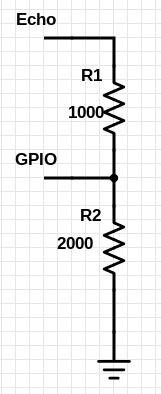Figure 4: Voltage Divider

We began our testing of the room measurement subsystem by setting up the distance sensor circuits. We initially tried using the level shifter as described above but found that distances were not being measured correctly. We then switched to the voltage divider and made sure the sensor worked by placing objects at varying distances from the sensor and checking if the distance reported was correct. Once we saw our distance sensor was functioning correctly, we integrated it onto the robot to test finding walls. We tested this by having the robot take forward steps until the distance sensor detected that an object was close. Once we saw that the two systems were working together we adjusted the distance threshold until we were satisfied that the robot moved close enough to the wall without bumping into it. After this tuning we had the distance measuring working correctly.

### Movement and Orientation

In order to move around the room we used two continuous rotation servos. These two servos worked by sending them pulses every 20 ms. How fast the servos rotated and in which direction depended on the width of the pulses sent. The two servos used were not consistent when set to the same speed and would sometimes jitter or not stop exactly at the same time. Because of this we needed a system to allow the robot to drive straight and to make any turns accurately. We decided to use the LSM303 accelerometer and magnetometer chip to handle these issues. The chip was capable of outputting how much it accelerated as well as the magnetic field in three axes. We decided to utilize the magnetometer rather than the accelerometer for navigation.

The LSM303 chip was capable of communicating using either I2C or SPI. We used the I2C interface because the SPI pins on our Pi were being used for interfacing with the TFT display. We decided to use Adafruit’s Python LSM303 library which handled reading magnetometer values off of the chip for us. We used the library to read off the two horizontal magnetic field values and took the inverse tangent of them in order to obtain a compass heading. At first we found that our headings were very inaccurate. After doing some research, we found that we needed to calibrate the chip as the earth’s magnetic field was not necessarily parallel to the ground unless we were at the equator. We began calibration by writing a program that would keep track of the maximum and minimum magnetometer readings as we rotated the chip around in all directions. We then used these values to calculate several intermediate values. The first value was rj = .5 * (maxj - minj) for j = x, y, z. The second value calculated was zj = maxj - rj for j = x,y,z. The calibrated results were then obtained by then subtracting the corresponding z from the sensor reading and dividing the result by the corresponding r.

We unfortunately found that there was a large amount of magnetic interference when attempting to use the magnetometer. The interference would alter the measured data so much that the robot would behave erratically. When moving, it would think the correct heading was in the wrong direction and attempt to adjust its heading to be in that wrong direction. We did not have time to create a new solution to the problem of the robot drifting and instead had to just adjust the speeds of the servos so the robot would move as straight as possible. We attempted to have the robot make accurate turns by running the motors at equal and opposite speeds for a set time, and tuned this time to what appeared to be accurate 90 degree turns.

For moving the robot forward or backwards, it was programmed to move in steps of about 5 inches, equal to the robot’s frame length. Once the robot reached a wall, it would turn around by first making a 90 degree turn, stepping forwards, and then making a second 90 degree turn in the same direction. By making the step 5 inches the robot would shift over by its width when it made the turn. We tuned the servo motor’s activation time to find the correct time needed to move the appropriate distance. We did not use a sensor to ensure the distance was exactly the same for each step, as making slightly short or long steps would not cause errors in operation. If the distance was incorrect the robot would either move through part of the same space twice or miss a small amount of space, both of which were considered acceptable.

We began testing the orientation systems by connecting the magnetometer and checking if the readings changed as we rotated the device. We saw that values were changing and moved on to calculating headings with the compass. We printed heading values to the terminal periodically and rotated the device to see if it calculated headings correctly. Once this was complete we integrated the sensor and movement systems. We did not perform testing on the servos as we had working code for controlling them from a previous project.

The navigation system began running after the size of the room had been determined and handled navigating around the entire room and stopping in the after every spot in the room had been visited. The navigation received the width and length of the room as well as the last turn that was made when checking the size of the room. The turn was used to determine whether the robot was beginning in the bottom right or top left corner when navigating the room. Navigation began using all of this information to create an arrayMap. The robot then turned away from the wall it was facing and began stepping forward. The robot would step forward length amount of time and would reach the opposite wall. Once there the robot would turn opposite to its last turn, step forward, and turn again. It would then be one column over in the room and could then begin moving down the length of the room again. It would repeat these steps width amount of times which caused it to move in a lawnmower type pattern shown in Figure 5 and end in the opposite corner to where it started.As the robot navigated through the space it would also update the arrayMap and the display to show where it had been so far. At the end of the run the entire screen would be completely filled.

We tested our navigation algorithm by combining it with our display subsystem. We gave the navigation algorithm and arrayMap width and length of a room and wrote a test that would move through the test room and display the state of the arrayMap each step. We then watched the display as the navigation algorithm ran and made sure it moved through the test room in the correct pattern and stopped once the entire map had been marked as visited.

We did not integrate the navigation system into the robot until all other systems were complete as it required all other parts of the robot to be working in order to run. Once we integrated it we tested it by placing the robot in various rooms and running it. We then watched to see if the robot would move to every spot in the room.

### Vacuum/Rolling Sweeper

We initially wanted a vacuum setup on our robot to collect the dirt and debris off the floor. Building a vacuum is quite simple; there is an intake pipe that leads to a debris bay, which then connects to an exhaust pipe with a fan at the end. The fan would be oriented such that the airflow causes a sucking pressure at the intake pipe. Ideally, the amount of air extracted from the piping system would be the equal to the amount entering. See Figure 6 for a simplified look on the guts of a vacuum cleaner. This rate of air is commonly measured in cubic feet per minute (CFM). Most household vacuums have around 100 CFM, giving them the ability to deep clean carpets, and operate on 120V household power. The RPi can only supply up to 5V of power to a device, which heavily decreases the power we can send to a fan. We needed to utilize a small fan for compactness, but small 5V fans are only available up to ~30 CFM. Also due the the the crudeness of the system, likely to have air leaks, we determined that this setup would not generate enough positive pressure to lift debris off a floor. The street sweeper design could have worked, by attaching a bristled dowel to a small motor, but the design and manufacturing steps were never realized.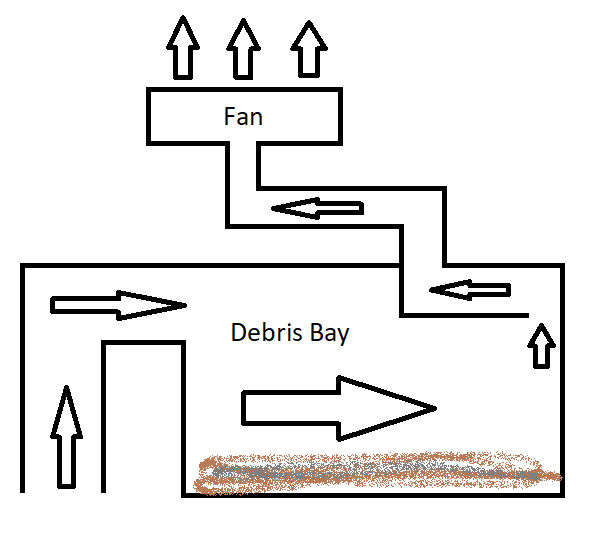Figure 6: Simple Vacuum Schematic

## Results

The finished project offered only a fraction of the functionality that was originally planned, however, what was presented in the final product function very well. The distance sensor detected walls as planned and the room dimensioning routine also worked in parallel with its function to create an appropriately sized grid on the TFT display. The TFT grid display updated correctly, corresponding to where the robot was in its current routine. The robot also accurately covered what it thought to be the entirety of the area it mapped. Although, since we hard coded all the turns and had no correction for forward movements, the additive error build up caused the robot to deviate off course. Despite deviation, the robot still completed the lawnmower movement scheme in its entirety with no wrong turns, and stopped when it finished mapping the room.

## Conclusion

We achieved a respectable room mapping robot with real time map updates via the TFT display. The robot moved in the lawnmower pattern as intended and stopped once if completed mapping the room. It also functioned entirely off of an embedded device, which was the main goal achievement of this project. Using a magnetometer to correct movement direction error and aid with rotational movements did not work due to magnetic field inconsistencies inside a building. The cleaning system needed more power output than our robot was capable of. Also hard-coding in movement steps and rotations worked, but were not accurate enough for the purpose of covering a room in its entirety.

## Code Appendix

main.py

 ``` 1 2 3 4 5 6 7 8 9 10 11 12 13 14 15 16 17 18 19 20 21 22 23 24 25 26 27 28 29 30 31 32 33 34 35 36 37 38 39 40 41 42 43 44 45 46 47 48 49 50 51 52 53 54 55 56 57 58 59 60 61 62 63 64 65 66 67 68 69 70 71 72 73 74 75 76 77 78 79 80 81 82 83 84 85 86 87 88 89 90 91 92 93 94 95 96 97 98 99 100 101 102 103 104 105 106 107 108 109 110 111 112 113 114 115 116 117 118 119 120 121 122 123 124 125 126 127 128 129 130 131 132 133 134 135 136 137 138 139 140 141 142 143 144 145 146 147 148 149``` ```from arrayMap import * from servoControl import * from distanceSensor import * import pygame # Import Library and initialize pygame import os import RPi.GPIO as GPIO import time from pygame.locals import * time.sleep(4) # setup TFT display os.putenv('SDL_VIDEODRIVER', 'fbcon') os.putenv('SDL_FBDEV', '/dev/fb1') os.putenv('SDL_MOUSEDRV', 'TSLIB') os.putenv('SDL_MOUSEDEV', '/dev/input/touchscreen') screen = pygame.display.set_mode(display_size) pygame.mouse.set_visible(False) try: #Setup quit button GPIO.setmode(GPIO.BCM) GPIO.setup(17, GPIO.IN, pull_up_down=GPIO.PUD_UP) def GPIO17_callback(channel): servo_cleanup() distanceCleanup() quit() GPIO.add_event_detect(17, GPIO.FALLING, callback=GPIO17_callback, bouncetime=300) display_size = display_width, display_height = 320,240 # screen size in pixels set_wall = 9 # distance threshold when checking for walls # setup subsystems pygame.init() pl, pr, lsm303 = servo_setup() distanceSetup() # find the length of the room sensor_dist = checkDistance() length = 0 # keep running until a wall is detected while(sensor_dist > set_wall): forwardStep2(pl,pr) sensor_dist = checkDistance() length = length + 1 #check for a wall to the right print('wall') turnRight(pl,pr) dist_check = checkDistance() last_turn = "right" time.sleep(0.5) # if there is a wall on the right turn around if(dist_check < (set_wall+2)): turnLeft(pl,pr) print('success') turnLeft(pl,pr) print('success') last_turn = "left" time.sleep(0.5) # find the width of the room width = 0 sensor_dist = checkDistance() print(str(sensor_dist)) print(str(dist_check)) while(sensor_dist > (set_wall)): print('width loop') forwardStep2(pl,pr) sensor_dist = checkDistance() width = width + 1 if(last_turn == 'left'): turnLeft(pl,pr) else: turnRight(pl,pr) # calculate grid values for use in display grid_width = display_width / width grid_height = display_height / length # decide initial position and direction based on last turn if(last_turn == "left"): curr_position = [0,0] curr_direction = 's' last_turn = "right" else: curr_position = [width-1,0] curr_direction = 's' last_turn = "left" # create arrayMap room_map = arrayMap(width,length,curr_position) displayMap(grid_width, grid_height, room_map,screen) # begin navigation for w in range(width-1): # move length steps forward for l in range(length-1): forwardStep2(pl,pr) # update map room_map.move(curr_direction,1) displayMap(grid_width, grid_height, room_map,screen) # turn opposite of last direction and move over one column if(last_turn == "left"): turnRight(pl,pr) last_turn = "right" else: turnLeft(pl,pr) last_turn = "left" curr_direction = rotateDirection(curr_direction, last_turn) forwardStep2(pl,pr) room_map.move(curr_direction,1) curr_direction = rotateDirection(curr_direction, last_turn) if(last_turn == "left"): turnLeft(pl,pr) else: turnRight(pl,pr) displayMap(grid_width, grid_height, room_map,screen) # should be in top right corner so need to move down length on last time for l in range(length): forwardStep2(pl,pr) # update map room_map.move(curr_direction,1) displayMap(grid_width, grid_height, room_map,screen) # stop and display the map until the quit button is pressed while True: displayMap(grid_width, grid_height, room_map,screen) # cleanup servo_cleanup() distanceCleanup() print('fin') quit() except KeyboardInterrupt: #do cleanup servo_cleanup() distanceCleanup() quit() ```

arrayMap.py

 ``` 1 2 3 4 5 6 7 8 9 10 11 12 13 14 15 16 17 18 19 20 21 22 23 24 25 26 27 28 29 30 31 32 33 34 35 36 37 38 39 40 41 42 43 44 45 46 47 48 49 50 51 52 53 54 55 56 57 58 59 60 61 62 63 64 65 66 67 68 69 70 71 72 73 74 75 76 77 78 79 80 81 82 83 84 85 86 87 88 89 90 91 92 93 94 95 96 97 98 99 100 101 102 103 104 105 106 107 108 109 110 111 112 113 114 115 116 117 118 119 120 121 122 123 124 125 126 127 128 129 130 131 132 133 134 135 136 137``` ```# Test using pygame gui to display path taken import pygame black = 0,0,0 white = 255,255,255 display_size = display_width, display_height = 320,240 #screen = pygame.display.set_mode(display_size) # Keeps track of the space the robot is navigating in and where it has been # if a cooridinate is False the space has not been visited # if a coordinate is True the space has been visited # position 0,0 is the top left most space in the map # position is of format [x][y] class arrayMap: # width = width of space in UNITS # length = length of space in UNITS def __init__(self, width, length, position): assert(isinstance(position, list)),"position is not a list" self.width = width # number of columns self.length = length # number of row #initialize map self.array_map = [[False]*width for _ in range(length)] self.position = position # access array in formate array_map[row][col] self.array_map[position][position] = True # Print the current map to the console def printMap(self): for i in range(self.length): print(self.array_map[i]) # move the current position a specified distance and mark spots as visited # inputs are 'n'orth, 's'outh, 'e'ast, and 'w'est # if distance would move off of map the movement stops at the edge def move(self, direction, dist): assert((direction == 'n') or (direction == 's') or (direction == 'e') or (direction == 'w')), "invalid direction" if(direction == 'n'): # update positions as visited for i in range(dist + 1): # check that maximum position is on map if((self.position - i) >= 0): self.array_map[self.position - i][self.position] = True if((self.position - dist) >= 0): self.position -= dist else: self.position = 0 elif(direction == 's'): for i in range(dist + 1): if((self.position + i) < self.length): self.array_map[self.position + i][self.position] = True if((self.position + dist) < self.length): self.position += dist else: self.position = self.length-1 elif(direction == 'e'): for i in range(dist + 1): if((self.position + i) < self.width): self.array_map[self.position][self.position + i] = True if((self.position + dist) < self.width): self.position += dist else: self.position = self.width - 1 elif(direction == 'w'): for i in range(dist + 1): if((self.position - i) >= 0): self.array_map[self.position][self.position - i] = True if((self.position - dist) >= 0): self.position -= dist else: self.position = 0 # mark a space as not visited def clearSpace(self, position): self.array_map[position][position] = False # mark a space as visited def setSpace(self, position): self.array_map[position][position] = True # reset entire map def clearMap(self): self.array_map = [[False]*self.width for _ in range(self.length)] # Rotate direction 90 degrees left or right # curr_dir is the current direction # turn_dir is left or right turn # returns the new direction def rotateDirection(curr_dir, turn_dir): assert((curr_dir == 'n') or (curr_dir == 's') or (curr_dir == 'e') or (curr_dir == 'w')), "invalid direction" assert(turn_dir == "left") or (turn_dir == "right"), "invalid turn direction" if(curr_dir == 'n'): if(turn_dir == "left"): return 'w' else: return 'e' elif(curr_dir == 'w'): if(turn_dir == "left"): return 's' else: return 'n' elif(curr_dir == 'e'): if(turn_dir == "left"): return 'n' else: return 's' elif(curr_dir == 's'): if(turn_dir == "left"): return 'e' else: return 'w' # Display the current state of an arrayMap # grid_width = width / display width # grid_height = length over display height # screen is the pygame screen object def displayMap(grid_width, grid_height, array_map, screen): screen.fill(black) for i in range(array_map.width): for j in range(array_map.length): x_pos = 0+i*grid_width y_pos = 0+j*grid_height outer_rect = pygame.Rect(x_pos, y_pos, grid_width, grid_height) inner_rect = pygame.Rect(x_pos+1, y_pos+1, grid_width-2, grid_height-2) color = white if(array_map.array_map[j][i]) else black pygame.draw.rect(screen, black, outer_rect) pygame.draw.rect(screen, color, inner_rect) pygame.display.flip() ```

distanceSensor.py

 ``` 1 2 3 4 5 6 7 8 9 10 11 12 13 14 15 16 17 18 19 20 21 22 23 24 25 26 27 28 29 30 31 32 33 34 35 36 37 38 39 40``` ```import RPi.GPIO as GPIO import time # setup sensor and GPIO pins def distanceSetup(): GPIO.setmode(GPIO.BCM) GPIO.setup(26, GPIO.OUT) GPIO.setup(19, GPIO.IN) GPIO.output(26,0) def checkDistance(): # send out 10 us pulse GPIO.output(26,1) time.sleep(0.00001) GPIO.output(26,0) # flag is used to ensure each while loop is entered correctly flag = 0 # wait for response pulse while(GPIO.input(19) == 0): start_time = time.time() flag = 1 # measure length of response pulse while(GPIO.input(19) == 1): end_time = time.time() flag = 2 # if something goes wrong return a large distance if(flag != 2): return 50 duration = end_time - start_time # conversion distance_inch = (duration * 1000000) / 148 #distance_cm = (duration * 1000000) / 58 return distance_inch # cleanup GPIO pins def distanceCleanup(): GPIO.cleanup() ```

servoControl.py

 ``` 1 2 3 4 5 6 7 8 9 10 11 12 13 14 15 16 17 18 19 20 21 22 23 24 25 26 27 28 29 30 31 32 33 34 35 36 37 38 39 40 41 42 43 44 45 46 47 48 49 50 51 52 53 54 55 56 57 58 59 60 61 62 63 64 65 66 67 68 69 70 71 72 73 74 75 76 77 78 79 80 81 82 83 84 85 86 87 88 89 90 91 92 93 94 95 96 97 98 99 100 101 102 103 104 105 106 107 108 109 110 111 112 113 114 115 116 117 118 119 120 121 122 123 124 125 126 127 128 129 130 131 132 133 134 135 136 137 138 139 140 141 142 143 144 145 146 147 148 149 150 151 152 153 154 155 156 157 158 159 160 161 162 163 164 165 166 167 168 169 170 171 172 173 174 175 176 177 178 179 180 181 182 183 184 185 186 187 188 189 190 191 192 193 194 195 196 197 198 199 200 201 202 203 204 205 206 207 208 209 210 211 212 213 214 215 216 217 218 219 220 221 222``` ```import RPi.GPIO as GPIO import time import Adafruit_LSM303.LSM303 import math # setup GPIO and magnetometer # return two pwm objects and an lsm303 object def servo_setup(): GPIO.setmode(GPIO.BCM) GPIO.setup(16, GPIO.OUT) GPIO.setup(20, GPIO.OUT) pl = GPIO.PWM(16,47) pl.start(0) pr = GPIO.PWM(20,47) pr.start(0) lsm303 = Adafruit_LSM303.LSM303() return pl, pr, lsm303 # set speed of servo # pulse_time is the length of the pulse to send to the servo # pwm_obj is the pwm_obj corresponding to the servo whose speed is being changed def set_speed(pulse_time, pwm_obj): p = pwm_obj valley = 20 #time between each pulse for motor servo control (msec) peak = pulse_time freq = 1/((valley+peak)*0.001) duty = peak/(valley+peak)*100 p.ChangeFrequency(freq) p.ChangeDutyCycle(duty) # stop a servo corresponding to pwm_obj def stop_motor(pwm_obj): p = pwm_obj p.ChangeFrequency(47) p.ChangeDutyCycle(0) # Given current magnetometer reading and target reading # returns speed adjustment needed # currently proportional term only def p_control(current, target): # proportional constant P = 0.004 # Integral constant #I = 0 # Derivative constant #D = 0 # calculate current error error = target - current #account for wrap around at 0 or 360 if(error > 180): error = error - 360 elif(error < -180): error = error + 360 # calculate amount to adjust speed adjustment = P * error # ensure amount will not result in servos spinning backwards if(adjustment < -0.1): adjustment = -0.1 return adjustment elif(adjustment > 0.1): adjustment = 0.1 return adjustment else: return adjustment # return heading determined by magnetometer def getDirection(lsm303): # calibration constants found earlier #maxX = 697 #minX = -514 maxY = 515 minY = -514 maxZ = 166 minZ = -638 # get magnetometer readings accel, mag = lsm303.read() mag_x,mag_y,mag_z = mag # calculate adjusted readings #rX = .5 * (maxX - minX) #zX = maxX - rX rY = .5 * (maxY - minY) zY = maxY - rY rZ = .5 * (maxZ - minZ) zZ = maxZ - rZ #mag_cal_X = (mag_x - zX) / rX mag_cal_Z = (mag_z - zZ) / rZ mag_cal_Y = (mag_y - zY) / rY # calculate heading mag_dir = (math.atan2(mag_cal_Y,mag_cal_Z) * 180) / 3.1415 if(mag_dir < 0): mag_dir = mag_dir + 360 return mag_dir # move the robot forward one step # NOTE: this function was unused due to problems with the magnetometer def reverseStep(pl,pr,lsm303,target): # get heading mag_dir = getDirection(lsm303) # get error correcting speed adjustment adjustment = p_control(mag_dir,target) left_speed = 1.4 right_speed = 1.6 set_speed(left_speed, pl) set_speed(right_speed, pr) start_time = time.time() curr_time = time.time() # run the motor long enough to move one step forward while((curr_time - start_time) < 0.75): # slow one of the wheel to correct error if(adjustment > 0): left_speed = 1.4 + adjustment else: right_speed = 1.6 + adjustment set_speed(left_speed, pl) set_speed(right_speed, pr) time.sleep(0.15) # get new reading mag_dir = getDirection(lsm303) adjustment = p_control(mag_dir,target) curr_time = time.time() stop_motor(pl) stop_motor(pr) # move the robot backwards one step # NOTE: this function was unused due to problems with the magnetometer def forwardStep(pl,pr,lsm303,target): mag_dir = getDirection(lsm303) adjustment = p_control(mag_dir,target) left_speed = 1.6 right_speed = 1.4 set_speed(left_speed, pl) set_speed(right_speed, pr) start_time = time.time() curr_time = time.time() while((curr_time - start_time) < 0.75): if(adjustment < 0): left_speed = 1.6 + adjustment right_speed = 1.4 else: right_speed = 1.4 + adjustment left_speed = 1.6 set_speed(left_speed, pl) set_speed(right_speed, pr) time.sleep(0.15) mag_dir = getDirection(lsm303) #print(str(mag_dir)) adjustment = p_control(mag_dir,target) curr_time = time.time() print(str(left_speed)+ ','+ str(right_speed)) print("targ = " + str(target) + ", curr = " + str(mag_dir) + ", adj = " + str(adjustment)) stop_motor(pl) stop_motor(pr) # move the robot backwards one step without using magnetometer def forwardStep2(pl,pr): left_speed = 1.6 right_speed = 1.415 set_speed(left_speed, pl) set_speed(right_speed, pr) start_time = time.time() curr_time = time.time() while((curr_time - start_time) < 0.75): curr_time = time.time() stop_motor(pl) stop_motor(pr) # turn the robot 90 degrees to the left (ccw) # returns target heading def turnRight(pl,pr): # make robot begin spinning left_speed = 1.6 right_speed = 1.6 set_speed(left_speed,pl) set_speed(right_speed,pr) start_time = time.time() curr_time = time.time() # spin 90 degrees while((curr_time-start_time) < 0.7): curr_time = time.time() stop_motor(pl) stop_motor(pr) # turn the robot 90 degrees to the right (cw) # returns target heading def turnLeft(pl,pr): left_speed = 1.45 right_speed = 1.45 set_speed(left_speed,pl) set_speed(right_speed,pr) start_time = time.time() curr_time = time.time() while((curr_time-start_time) < 0.85): curr_time = time.time() stop_motor(pl) stop_motor(pr) # cleanup gpio pins def servo_cleanup(): GPIO.cleanup() ```

Distance Sensor -

https://www.sparkfun.com/products/13959

Accelerometer and Magnetometer -

## Parts List and Budget

Part Name Part Number Quantity Cost Total Cost Link
Servos   2 \$10.00 \$20.00 http://a.co/0BU8GHI
Robot Frame   1 \$0.00 \$0.00
Magnetometer LSM303 1 \$3.94 \$3.94 https://www.adafruit.com/product/1120
Distance Sensor HC-SR04 1 \$14.95 \$14.95 https://www.sparkfun.com/products/13959
RPi Kit   1 \$0.00 \$0.00
Grand Total       \$38.89

## Work Division

### Douglas Katz

• Wrote servoControl code
• Wrote distanceSensor code
• Wrote navigation / main code
• Assembled distanceSensor circuit
• Assembled magnetometer circuit
• Tested servoControl code
• Tested distanceSensor code
• Tested arrayMap code
• Tested navigation / main code
• Wrote objective, introduction, and design and testing sections of report
• Formatted report into website

### Greg Cristina

• Robot Design and Vacuum Trade Study
• Wrote servoControl code
• Wrote navigation / main code
• Debugged/Tested magnetometer
• Tested servoControl
• Tested navigation / main code
• Tested distanceSensor
• Wrote design and testing, results, conclusion sections of report
• Some website formatting